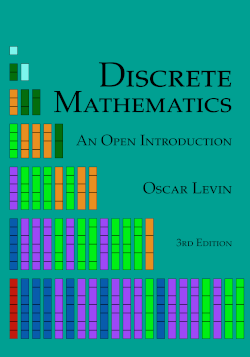# Discrete Mathematics: An Open Introduction, 3rd edition

## Section0.1What is Discrete Mathematics?

dis·crete / dis’krët.
Synonyms: separate - detached - distinct - abstract.
Defining discrete mathematics is hard because defining mathematics is hard. What is mathematics? The study of numbers? In part, but you also study functions and lines and triangles and parallelepipeds and vectors and …. Or perhaps you want to say that mathematics is a collection of tools that allow you to solve problems. What sort of problems? Okay, those that involve numbers, functions, lines, triangles, …. Whatever your conception of what mathematics is, try applying the concept of “discrete” to it, as defined above. Some math fundamentally deals with stuff that is individually separate and distinct.
In an algebra or calculus class, you might have found a particular set of numbers (maybe the set of numbers in the range of a function). You would represent this set as an interval: $$[0,\infty)$$ is the range of $$f(x) = x^2$$ since the set of outputs of the function are all real numbers 0 and greater. This set of numbers is NOT discrete. The numbers in the set are not separated by much at all. In fact, take any two numbers in the set and there are infinitely many more between them which are also in the set.
Discrete math could still ask about the range of a function, but the set would not be an interval. Consider the function which gives the number of children of each person reading this. What is the range? I’m guessing it is something like $$\{0, 1, 2, 3\}\text{.}$$ Maybe 4 is in there too. But certainly there is nobody reading this that has 1.32419 children. This output set is discrete because the elements are separate. The inputs to the function also form a discrete set because each input is an individual person.
One way to get a feel for the subject is to consider the types of problems you solve in discrete math. Here are a few simple examples:
###### Investigate!
Note: Throughout the text you will see Investigate! activities like this one. Answer the questions in these as best you can to give yourself a feel for what is coming next.
1. The most popular mathematician in the world is throwing a party for all of his friends. As a way to kick things off, they decide that everyone should shake hands. Assuming all 10 people at the party each shake hands with every other person (but not themselves, obviously) exactly once, how many handshakes take place?
2. At the warm-up event for Oscar’s All Star Hot Dog Eating Contest, Al ate one hot dog. Bob then showed him up by eating three hot dogs. Not to be outdone, Carl ate five. This continued with each contestant eating two more hot dogs than the previous contestant. How many hot dogs did Zeno (the 26th and final contestant) eat? How many hot dogs were eaten all together?
3. After excavating for weeks, you finally arrive at the burial chamber. The room is empty except for two large chests. On each is carved a message (strangely in English):
If this chest is empty, then the other chest’s message is true.
This chest is filled with treasure or the other chest contains deadly scorpions.
You know exactly one of these messages is true. What should you do?
4. Back in the days of yore, five small towns decided they wanted to build roads directly connecting each pair of towns. While the towns had plenty of money to build roads as long and as winding as they wished, it was very important that the roads not intersect with each other (as stop signs had not yet been invented). Also, tunnels and bridges were not allowed. Is it possible for each of these towns to build a road to each of the four other towns without creating any intersections?
One reason it is difficult to define discrete math is that it is a very broad description which encapsulates a large number of subjects. In this course we will study four main topics: combinatorics (the theory of ways things combine; in particular, how to count these ways), sequences, symbolic logic, and graph theory. However, there are other topics that belong under the discrete umbrella, including computer science, abstract algebra, number theory, game theory, probability, and geometry (some of these, particularly the last two, have both discrete and non-discrete variants).
Ultimately the best way to learn what discrete math is about is to do it. Let’s get started! Before we can begin answering more complicated (and fun) problems, we must lay down some foundation. We start by reviewing mathematical statements, sets, and functions in the framework of discrete mathematics.